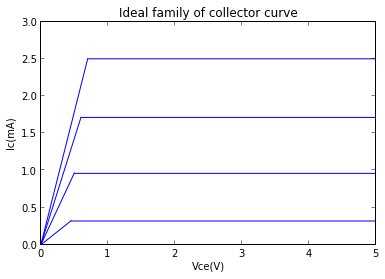Chapter 4: Bipolar Junction Transistors (BJTs)

Example 4.1, Page Number: 120

In :
%matplotlib inline
Welcome to pylab, a matplotlib-based Python environment [backend: module://IPython.zmq.pylab.backend_inline].
In :
# variable declaration
I_C=3.65*10**-3;    #collector current in amperes
I_B=50*10**-6;    #base current in amperes

#calculation
B_DC=I_C/I_B;  #B_DC value
I_E=I_B+I_C;   #current in ampere

# result
print "B_DC = %d " %B_DC
print "Emitter current = %.4f ampere" %I_E
B_DC = 73
Emitter current = 0.0037 ampere

Example 4.2, Page Number: 121

In :
# variable declaration
V_BE=0.7;         # voltage in volt
B_DC=150;         # voltage in volt
V_BB=5;           # voltage in volt
V_CC=10;          # voltage in volt
R_B=10*10**3;      # resistance in ohm
R_C=100;          # resistance in ohm

#calculation
I_B=(V_BB-V_BE)/R_B;  #base current in amperes
I_C=B_DC*I_B;         #collector current in amperes
I_E=I_C+I_B;          #emitter current in amperes
V_CE=V_CC-I_C*R_C;    #collector to emitter voltage in volts
V_CB=V_CE-V_BE;       #collector to base voltage in volts

# result
print "base current = %.5f amperes" %I_B
print "collector current = %.4f amperes" %I_C
print "emitter current = %.5f amperes" %I_E
print "collector to emitter voltage =%.2f volts" %V_CE
print "collector to base voltage =%.2f volts" %V_CB
base current = 0.00043 amperes
collector current = 0.0645 amperes
emitter current = 0.06493 amperes
collector to emitter voltage =3.55 volts
collector to base voltage =2.85 volts

Example 4.3, Page Number: 123

In :
import pylab as py
import numpy as np

#variable declaration
beta=100 # current gain
print'Ideal family of collector curve'

ic1 = arange(0.00001, 0.45, 0.0005)
ic2 = arange(0.00001, 0.5, 0.0005)
ic3 = arange(0.00001, 0.6, 0.0005)
ic4 = arange(0.00001, 0.7, 0.0005)
vcc1=ic1*0.5/0.7
vcc2=ic2*1.35/0.7
vcc3=ic3*2/0.7
vcc4=ic4*2.5/0.7
m1=arange(0.45,5.0,0.0005)
m2=arange(0.5,5.0,0.0005)
m3=arange(0.6,5.0,0.0005)
m4=arange(0.7,5.0,0.0005)

plot(ic1,vcc1,'b')
plot(ic2,vcc2,'b')
plot(ic3,vcc3,'b')
plot(ic4,vcc4,'b')
plot(m1,0.32*m1/m1,'b')
plot(m2,0.96*m2/m2,'b')
plot(m3,1.712*m3/m3,'b')
plot(m4,2.5*m4/m4,'b')

ylim( (0,3) )
ylabel('Ic(mA)')
xlabel('Vce(V)')
title('Ideal family of collector curve')
Ideal family of collector curve
Out:
<matplotlib.text.Text at 0xa11e74c>Example 4.4, Page Number: 125

In :
# variable declaration
V_CE_sat=0.2;  # voltage in volt
V_BE=0.7;      # voltage in volt
V_BB=3;        # voltage in volt
V_CC=10;       # voltage in volt
B_DC=50;       # voltage in volt
R_B=10*10**3;   # resistance in ohm
R_C=1*10**3;     # resistance in ohm

#calculation
I_C_sat=(V_CC-V_CE_sat)/R_C;   # saturation current
I_B=(V_BB-V_BE)/R_B;           # base current
I_C=B_DC*I_B;                  # current in ampere

# result
if I_C>I_C_sat:
print "transistor in saturation"
else:
print "transistor not in saturation"
transistor in saturation

Example 4.5, Page Number: 127

In :
#Variable declaration
P_D_max=250*10**-3;    #max power rating of transistor in watts
V_CE=6;                #voltage in volt

#Calculation
I_Cu=P_D_max/V_CE;        #Current (Amp)
I_C=I_Cu*1000;

#Result
print "collector current that can be handled by the transistor = %.1f mA" %I_C
print "\nRemember that this is not necessarily the maximum IC. The transistor"
print "can handle more collectore current if Vce is reduced as long as PDmax"
print "is not exceeded."
collector current that can be handled by the transistor = 41.7 mA

Remember that this is not necessarily the maximum IC. The transistor
can handle more collectore current if Vce is reduced as long as PDmax
is not exceeded.

Example 4.6, Page Number: 127

In :
#Variable declaration
P_D_max=800*10**-3;    #max power rating of transistor in watts
V_BE=0.7;             #voltage in volt
V_CE_max=15;          #voltage in volt
I_C_max=100*10**-3;    #Current (Amp)
V_BB=5;               #voltage in volt
B_DC=100;             #voltage in volt
R_B=22*10**3;          # resistance in ohm
R_C=10**3;             # resistance in ohm

#Calculation
I_B=(V_BB-V_BE)/R_B;  # base current
I_C=B_DC*I_B;         #collector current
V_R_C=I_C*R_C;        #voltage drop across R_C
V_CC_max=V_CE_max+V_R_C; #Vcc max in volt
P_D=I_C*V_CE_max;       #max power rating

#Result
if P_D<P_D_max:
print "V_CC = %.2f volt" %V_CC_max
print "V_CE_max will be exceeded first because entire supply voltage V_CC will be dropped across the transistor"

V_CC = 34.55 volt
V_CE_max will be exceeded first because entire supply voltage V_CC will be dropped across the transistor

Example 4.7, Page Number: 128

In :
#Variable declaration
df=5*10**-3;    #derating factor in watts per degree celsius
T1=70;         #temperature 1
T2=25;         #temperature 2
P_D_max=1;    #in watts

#Calculation
del_P_D=df*(T1-T2);   #change due to temperature
P_D=P_D_max-del_P_D;  # power dissipation

#Result
print "Power dissipated max at a temperature of 70 degree celsius = %.3f watts" %P_D
Power dissipated max at a temperature of 70 degree celsius = 0.775 watts

Example 4.8, Page Number: 130

In :
#Variable declaration
R_C=1*10**3;  #resistance in ohm
r_e=50;      #resistance in ohm
V_b=100*10**-3; #voltage in volt

#Calculation
A_v=R_C/r_e;   #voltage gain
V_out=A_v*V_b; #voltage in volt

#Result
print "voltage gain = %d " %A_v
print "AC output voltage = %d volt" %V_out
voltage gain = 20
AC output voltage = 2 volt

Example 4.9, Page Number: 132

In :
#Variable declaration
V_CC=10.0;   #voltage in volt
B_DC=200.0;  #voltage in volt
R_C=1.0*10**3;  #resistance in ohm
V_IN=0.0;    #voltage in volt

#Calculation
V_CE=V_CC;   #equal voltage
print "when V_IN=0, transistor acts as open switch(cut-off) and collector emitter voltage = %.2f volt" %V_CE
#now when V_CE_sat is neglected
I_C_sat=V_CC/R_C; #saturation current
I_B_min=I_C_sat/B_DC;  #minimum base current
print "\nminimum value of base current to saturate transistor = %.5f ampere" %I_B_min
V_IN=5;     #voltage in volt
V_BE=0.7;   #voltage in volt
V_R_B=V_IN-V_BE;    #voltage across base resiatance
R_B_max=V_R_B/I_B_min;

#Result
kw=round (R_B_max)
print "\nmaximum value of base resistance when input voltage is 5V = %d ohm" %kw
when V_IN=0, transistor acts as open switch(cut-off) and collector emitter voltage = 10.00 volt

minimum value of base current to saturate transistor = 0.00005 ampere

maximum value of base resistance when input voltage is 5V = 86000 ohm